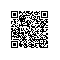# 2.3. Functions (函数)

### 2.3.1. 匿名函数（Anonymous functions）

#### 匿名函数（Anonymous functions）也叫闭包函数（closures）允许 临时创建一个没有指定名称的函数。


<?php
$put = function($name)
{
printf("%s\r\n", $name); };$put('World');
$put('PHP'); ?>   <?php$aaa = 111;
$func = function() use($aaa){ print $aaa; };$aaa = 222;
\$func(); // Outputs "111"
?>使用钉钉扫一扫加入圈子
+ 订阅